Refer to our Texas Go Math Grade 4 Answer Key Pdf to score good marks in the exams. Test yourself by practicing the problems from Texas Go Math Grade 4 Lesson 6.4 Answer Key Add Decimals.

Essential Question

In order to keep the numbers in the proper place-value column when adding decimals, align the decimal points. This will keep the numbers aligned; ones to ones, tenths to tenths, hundredths to hundredths, and so on.

Unlock the Problem

Henry recorded the amount of rain that fell over 2 hours. In the first hour, Henry measured 2.35 centimeters of rain. In the second hour, he measured 1.82 centimeters of rain.

Henry estimated that about 4 centimeters of rain fell in 2 hours What is the total amount of rain that fell? How can you use this estimate to decide if your answer is reasonable?

5 hundredths + 2 hundredths = ______________ hundredths.
5 hundredths + 2 hundredths = 7 hundredths.

• Then add the tenths and ones. Regroup as needed.• Record the sum for each place value.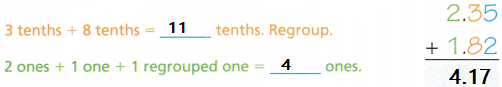Draw a quick picture to check your work.

So, ___________ centimeters of rain fell.
Since ___________ is close to the estimate, 4, the answer is reasonable.
So, 4.17 centimeters of rain fell.
Since 4.17 is close to the estimate, 4, the answer is reasonable.

Math Talk

Mathematical Processes
Explain how you know when you need to regroup in a decimal addition problem.
For decimals, we line up the decimal points so that the whole number parts line up and the decimal parts line up. Then add each. To add decimal numbers follow the step below. … Place the decimal point of the result in line with the other decimal points.

Equivalent Decimals When adding decimals, you can use equivalent decimals to help keep the numbers aligned in each place. Add zeros to the right of the last digit as needed, so that the addends have the same number of decimal places.

Try This! Estimate. Then find the sum.

STEP 1

Estimate the sum.

Estimate: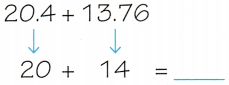20.40 + 13.76 = _____________
20.40 + 13.76 = 34.16

STEP 2

Find the sum.

Add the hundredths first. Then, add the tenths, ones, and tens. Regroup as needed.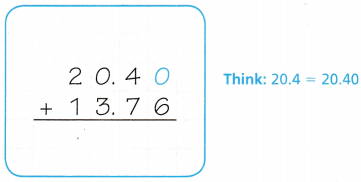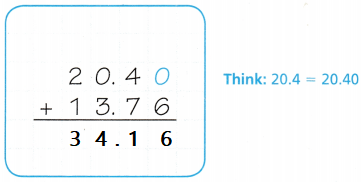Answer: yes, 34 is close to 34.16 so my answer is reasonable.

Share and Show

Estimate. Then find the sum.

Question 1.
Estimate: _________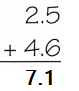Explanation:
Estimation of values 2 + 4 = 6

Question 2.
Estimate: _________Explanation:
Estimation of values 8 + 6 = 14

Question 3.
Estimate: _________Explanation:
Estimation of values 7 + 2 = 9

Question 4.
Estimate: _________
6.34 + 3.8 = _________
6.34 + 3.8 = 10.14
Explanation:
Estimation of values 6 + 3 = 9

Question 5.
Estimate: _________
0.63 + 0.6 = _________
0.63 + 0.6 = 1.23
Explanation:
Estimation of values 0 + 0 = 0

Math Talk

Mathematical Processes
Explain why it is important to remember to line up the place values in each number when adding or subtracting decimals.

Problem Solving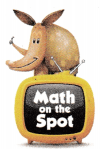Question 6.
H.O.T. Multi-Step A city received 4.65 centimeters of rain in the first two weeks of August. During the rest of the month it rained 3.68 centimeters more than it had the first two weeks. What is the total rainfall in centimeters for the month of August?
(A) 8.33 centimeters
(B) 12.01 centimeters
(C) 12.98 centimeters
(D) 11.99 centimeters
Explanation:
4.65 + 3.68 = 8.33
8.33 + 4.65 = 12.98 centimeters

a. What do you need to find?
Rainfall in centimeters for the month of August

b. What information are you given?
4.65 centimeters of rain in the first two weeks of August.
3.68 centimeters more than it had the first two weeks

C. How will you use addition to find the total number of centimeters of rain that fell?
4.65 + 3.68 = 8.33

d. Show how you soLved the problem.
4.65 + 3.68 = 8.33
8.33 + 4.65 = 12.98 centimeters

e. Fill in the bubble for the correct answer choice above.
Filled the correct bubble in the above options.

Question 7.
Apply Tania measured the growth of her plant each week. The first week, the plant’s height measured 2.65 decimeters. During the second week, Tania’s plant grew 0.38 decimeter. How tall was Tania’s plant at the end of the second week?
(A) 2.27 decimeters
(B) 3.03 decimeters
(C) 3.23 decimeters
(D) 3.93 decimeters
Explanation:
2.65 + 0.38 = 3.03 tall was Tania’s plant at the end of the second week

Question 8.
Multi-Step Maggie had $35.13. Then her mom gave her$7.50 each of the two times she watched her younger brother. how much money does Maggie have now?
(A) $41.63 (B)$42.63
(C) $41.63 (D)$50.13
Explanation:
35.13 + 7.50  + 7.50 = 50.13
50.13 money that Maggie have now.

Question 9.
A king’s crown has a ruby that is 4.9 carats and a sapphire that is 5.32 carats. What is the total number of carats on the king’s crown?
(A) 9.41 carats
(B) 9.22 carats
(C) 10.22 carats
(D) 11.12carats
Explanation:
4.9 + 5.32 = 10.22
10.22 is the total number of carats on the king’s crown.

Question 10.
10. Josh bought 1.75 pounds of apples and 2.4 pounds of oranges.
How many pounds of apples and oranges did he buy in all?
(A) 2.15 pounds
(B) 4.15 pounds
(C) 1.99 pounds
(D) 3.75 pounds
Explanation:
1.75 + 2.4 = 4.15
4.15 pounds of apples and oranges that they buy in all

Question 11.
Multi-Step Evaluate Sean runs 2.62 miles. Lisa runs 3.02 miles. Shannon runs 2.78 miles. Max runs 3.8 miles. Which two people run for a total of 5.4 miles?
(A) Sean and Shannon
(B) Lisa and Max
(C) Lisa and Shannon
(D) Sean and Max
Explanation:
2.62 + 3.02  = 5.64
2.62 + 2.78  =  5.4 Sean and Shannon run for a total of 5.4 miles

TEXAS Test Prep

Question 12.
When Mandy got a puppy, it weighed three and eighty-five hundredths pounds. After a month, it weighed ninety-five hundredths more than when she got it. How much did the puppy weigh at the end of the month?
(A) 480 pounds
(B) 4.70 pounds
(D) 4.80 pounds
(D) 3.70 pounds
Explanation:
3.85 + 0.95 = 4.8
4.80 pounds the puppy weigh at the end of the month

### Texas Go Math Grade 4 Lesson 6.4 Homework and Practice Answer Key

Estimate. The find the sum.

Question 1.
Estimate: ___________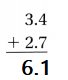Explanation:
Estimation of values 3 + 2 = 5

Question 2.
Estimate: ___________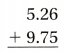Explanation:
Estimation of values 5 + 9 = 14

Question 3.
Estimate: ___________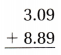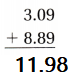Explanation:
Estimation of values 3 + 8 = 11

Question 4.
Estimate: ___________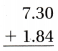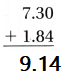Explanation:
Estimation of values 7 + 1 = 8

Question 5.
Estimate: ___________
5.29 + 6.78 = ___________
5.29 + 6.78 = 12.07
Explanation:
Estimation of values 5 + 6 = 11

Question 6.
Estimate: ___________
6.2 + 2.36 = ___________
6.2 + 2.36 = 8.56
Explanation:
Estimation of values 6 + 2 = 8

Question 7.
Estimate: ___________
9.2 + 3.04 = ___________
9.2 + 3.04 = 12.24
Explanation:
Estimation of values 9 + 3 = 12

Question 8.
Estimate: ___________
7.08 + 2.9 = ___________
7.08 + 2.9 = 9.98
Explanation:
Estimation of values 7 + 2 = 9

Question 9.
Estimate: ___________
7.86 + 2.9 = __________
7.86 + 2.9 = 10.76
Explanation:
Estimation of values 7 + 2 = 9

Question 10.
Estimate: ___________
4.3 + 2.49 = ___________
4.3 + 2.49 = 6.79
Explanation:
Estimation of values 4 + 2 = 6

Problem Solving

Question 11.
Monique’s dog weighs 10.6 pounds. Her cat weighs 8.4 pounds. What is the combined total weight of Monique’s pets?
10.6 + 8.4  = 19
Explanation:
19 is the combined total weight of Monique’s pets

Question 12.
Monique spent $8.49 on dog food and$7.99 on cat food. What is the total amount Monique spent on food for her pets?
8.49 + 7.99  = 16.48
Explanation:
16.48 is the total amount Monique spent on food for her pets

Lesson Check

Question 13.
Joseph ran 3.75 miles around the track. After resting, Joseph ran an additional 2.9 miles. What is the total distance Joseph ran?
(A) 3.94 miles
(B) 6.65 miles
(C) 4.04 miles
(D) 5.65 miles
Explanation:
3.75 + 2.9 = 6.65 is the total distance Joseph ran

Question 14.
On the first day of school, Cassandra measured 1.37 meters tall. During the school year, Cassandra grew 0.06 meters. How tall was Cassandra at the end of the school year?
(A) 1.33 meters
(B) 1.31 meters
(C) 1.43 meters
(D) 1.42 meters
Explanation:
1.37 + 0.06 = 1.43 tall was Cassandra at the end of the school year

Question 15.
Walter measured the growth of a plant for his science project. At the end of the first week, the plant was 9.54 centimeters tall. During the second week, the plant grew 2.68 centimeters. How tall was Walter’s plant at the end of the second week?
(A) 11.22 centimeters
(B) 12.12 centimeters
(C) 11.12 centimeters
(D) 12.22 centimeters
Explanation:
9.54 + 2.68  = 12.22 tall was Walter’s plant at the end of the second week

Question 16.
The distance from Jeff’s house to his uncle’s house is 12.58 miles. If Jeff travels another 9.49 miles, he will reach his grandmother’s house. What is the distance from Jeff’s house to his grand mother’s house?
(A) 22.07 miles
(B) 21.97 miles
(C) 21.07 miles
(D) 22.08 miles
Explanation:
12.58 + 9.49  = 22.07 is the distance from Jeff’s house to his grand mother’s house.

Question 17.
Multi-Step Kyle earned $9.50 per week for two weeks in a row helping his brother mow lawns. His brother paid him an additional$3.75 to clean the mower. What is the total amount Kyle earned?
(A) $12.75 (B)$19.00
(C) $22.75 (D)$13.25
Explanation:
9.50 + 9.50  + 3.75 = 22.75 is the total amount Kyle earned

Question 18.
Multi-Step Mrs. McDonald spent $24.65 at a yard sale on Saturday. On Sunday, she went to another yard sale and spent$14.95 more than she did on Saturday. What is the total amount that Mrs. McDonald spent at both yard sales?
(A) $38.60 (B)$64.25
(C) $39.60 (D)$63.25